﻿ 基于负荷转移模型的配网合环可行性研究 The Feasibility Research on Distribution Network Closed Loop Based on the Load Transfer Model

Journal of Electrical Engineering
Vol.05 No.02(2017), Article ID:20867,10 pages
10.12677/JEE.2017.52018

The Feasibility Research on Distribution Network Closed Loop Based on the Load Transfer Model

Qi Yin1, Rui Ding1, Yongchun Zhao2, Yanfen Liu2, Xiaolin Liu3

1State Grid Deyang Electric Power Supply Company, Deyang Sichuan

2Beijing Join Bright Digital Power Technology Co., Ltd., Beijing

3State Grid (Beijing) Energy Conservation Design and Research Institute Co. Ltd., Beijing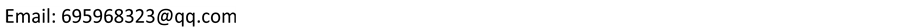Received: May 17th, 2017; accepted: Jun. 3rd, 2017; published: Jun. 6th, 2017ABSTRACT

In order to improve the success rate of power distribution without switching power distribution, this paper presents mathematical model of load transfer in distribution network. The differences of voltage of amplitude and phase angle on both sides are calculated by the power flow. According to the size of the difference, we can determine whether the operation can be carried out, thereby improving the success rate of operation.

Keywords:Power Flow, Surge Current, Closing Loop

1国网四川省电力公司德阳供电公司，四川 德阳

2北京中恒博瑞数字电力科技有限公司，北京

3国网(北京)节能设计研究院有限公司，北京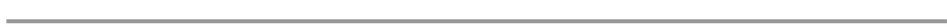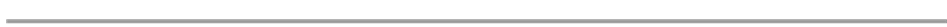1. 引言

2. 配网合环原理

2.1. 城市配电网常用结构

2.2. 负荷模型Figure 1. The diagram of 220 kV different network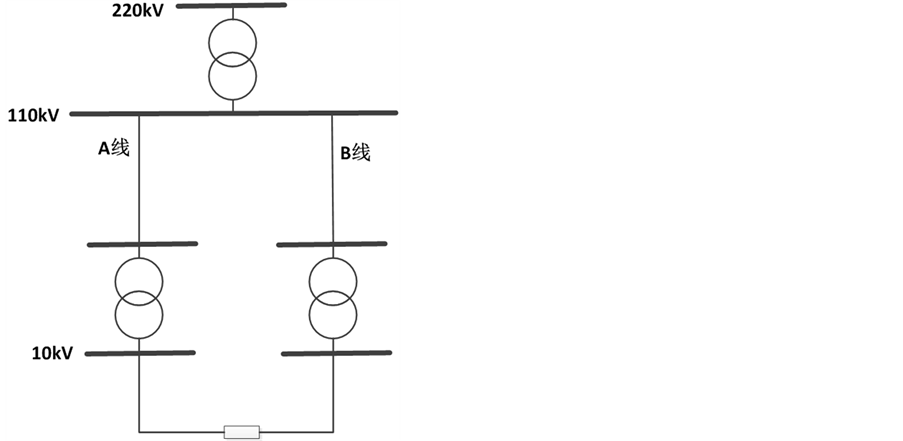(a) (b)

Figure 2. The diagram of 220 kV network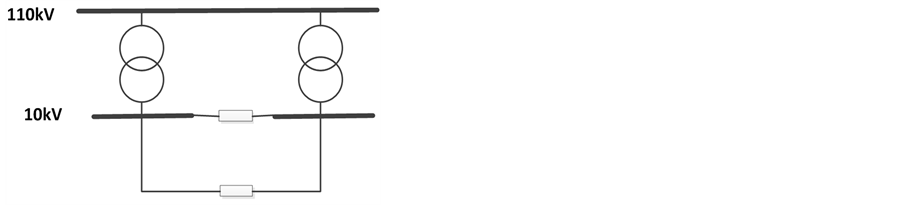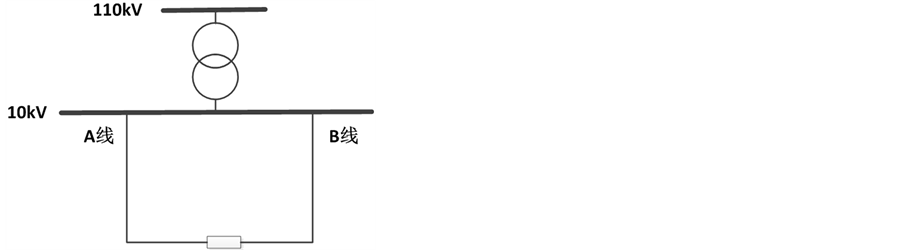(a) (b)

Figure 3. The diagram of the same plant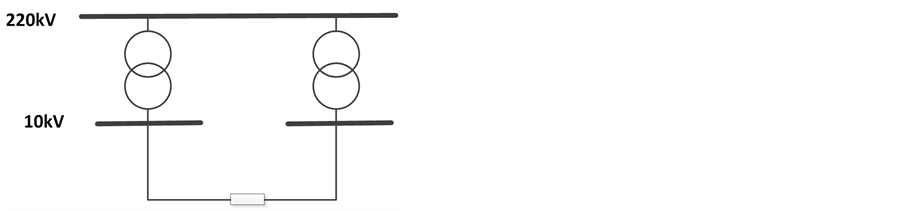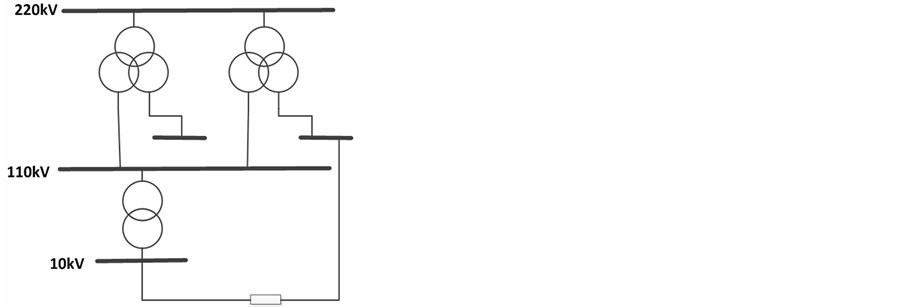(a) (b)

Figure 4. The diagram of the below of 220 kV main transformer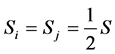(1)(2)

2.3. 配网合环判据(3)

2.4. 合环冲击电流模型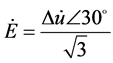(4)(5)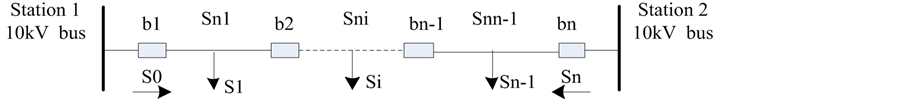Figure 5. The load model of distribution networkFigure 6. The diagram of closed loop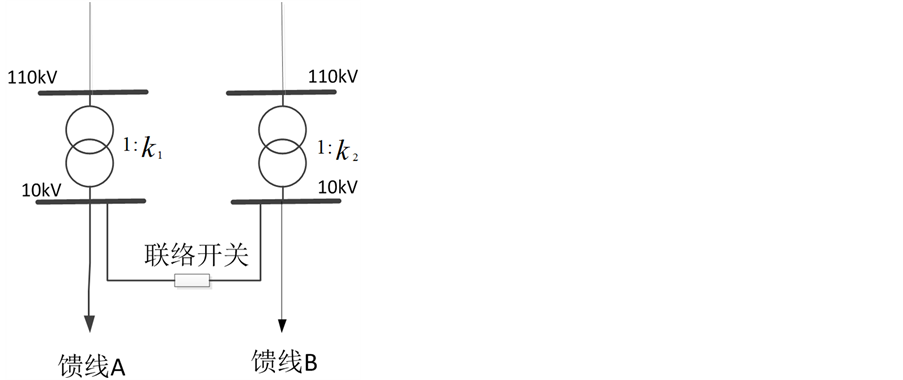Figure 7. The closed loop of network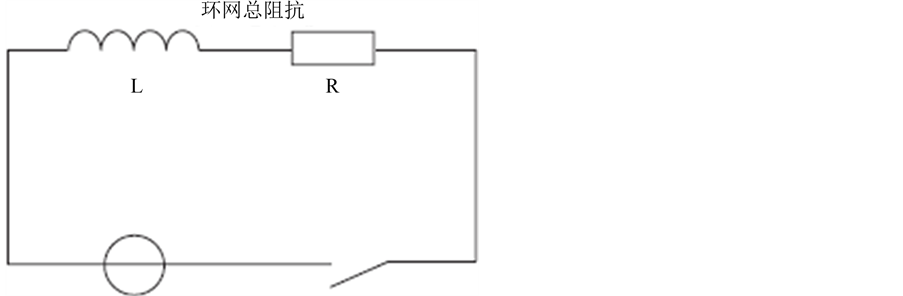Figure 8. The diagram of Davidon equivalent circuit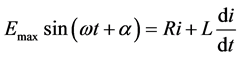(6)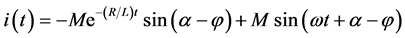(7)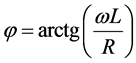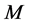为合环电流周期分量的幅值，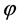为周期分量和间的相角。3. 潮流计算(8)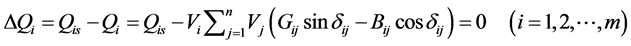(9)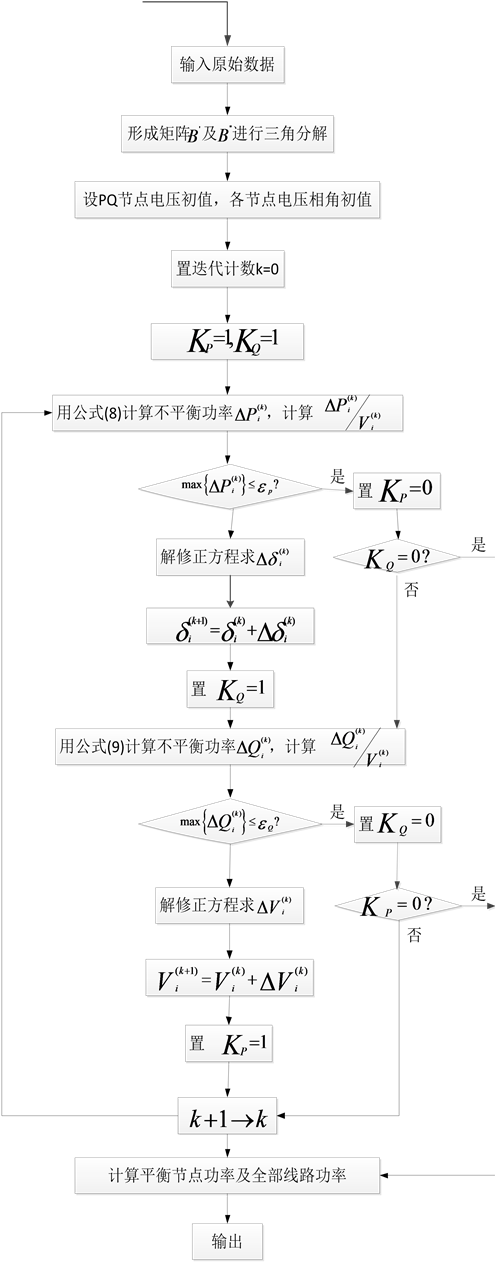Figure 9. The diagram of P-Q power flow

4. 算例分析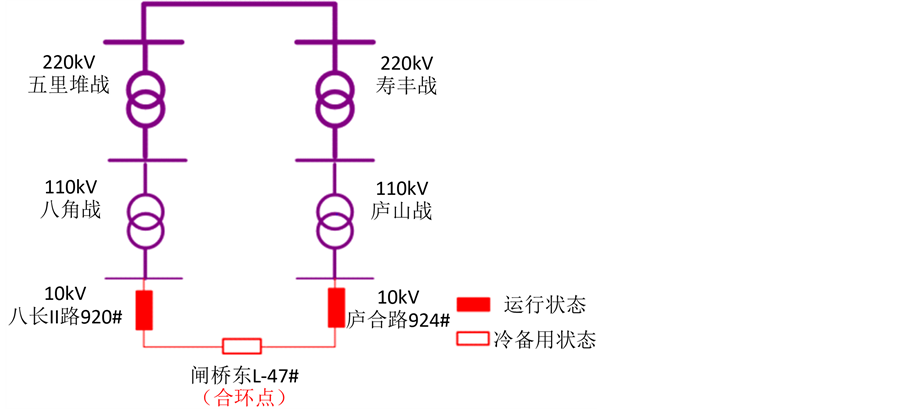Figure 10. The diagram of Typical wiring of Deyang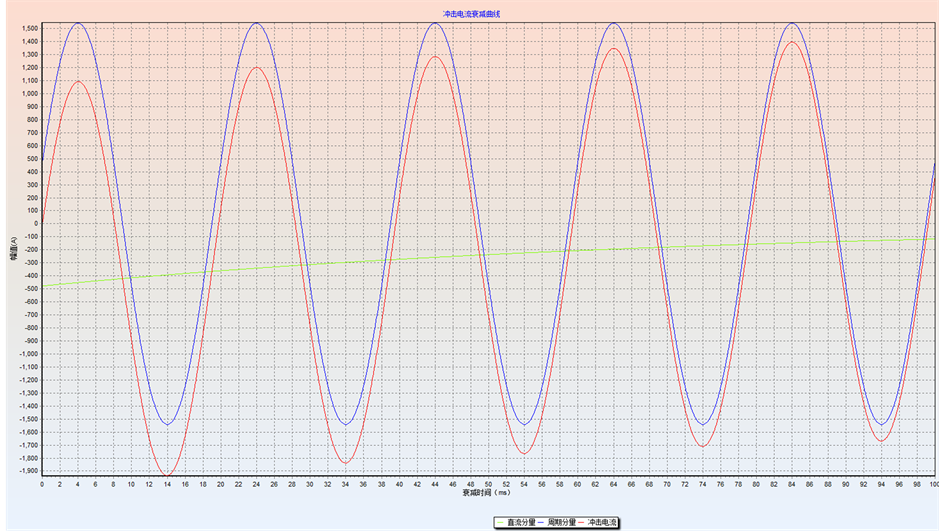Figure 11. The diagram of surge current of closed loopFigure 12. The block diagram of closed loop measurementTable 1. The information of current of closed loop of ZhaqiaodongTable 2. The information of voltage of closed loop of ZhaqiaodongTable 3. The information of voltage is obtained by measuring

5. 结论

The Feasibility Research on Distribution Network Closed Loop Based on the Load Transfer Model[J]. 电气工程, 2017, 05(02): 150-159. http://dx.doi.org/10.12677/JEE.2017.52018

1. 1. 刘磊, 禹化然, 金鑫. 合环潮流的暂态过程分析[J]. 工程机电, 2008, 25(10): 74-76.

2. 2. 李丛林. 天津电网潮流分析和暂态稳定仿真研究[D]: [硕士学位论文]. 天津: 天津大学, 2011.

3. 3. 李晓柯, 李祥发. 配电网合环冲击电流的暂态过程分析与仿真[J]. 机电工程, 2010, 27(5): 67.

4. 4. 夏翔, 熊军, 胡列翔. 地区电网的合环潮流分析与控制[J]. 电网技术, 2004, 28(22): 76-80.

5. 5. 陈成功, 杨昀, 李爱元, 凌志勇. 典型配电网合环潮流计算与分析[J]. 湖南电力, 2015, 35(4): 18-23, 26.

6. 6. 国家电网公司. 国家电网公司电力系统电压质量和无功电力管理规定[Z]. 2009: 2.

7. 7. 吕彬林, 刘敏, 徐天福. 快速解耦法潮流计算方法的比较[J]. 江西电力, 2013, 37(5): 67-69, 89.

8. 8. 何仰赞, 温增银. 电力系统分析[M]. 武汉: 华中科技大学出版社, 2010.

9. 9. 张兰秀. 合环操作在10kV配网中的应用探讨[EB/OL]. http://www.chinaqking.com/yc/2012/254556.html, 2012-8-23.

10. 10. 陈宵, 王磊, 李杨. 配电网络合环冲击电流的分析[J]. 电力自动化设备, 2005, 25(4): 40-42.

11. 11. 邱关源. 电路[M]. 北京: 高等教育出版社, 1999.